NCERT Solutions for Class 9th: Ch 6 Lines and Angles Maths

NCERT Solutions for Class 9th: Ch 6 Lines and Angles Maths

Page No: 96

Exercise 6.1

1. In Fig. 6.13, lines AB and CD intersect at O. If ∠AOC + ∠BOE = 70° and ∠BOD = 40°, find ∠BOE and reflex ∠COE.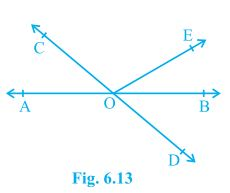Given,
∠AOC + ∠BOE = 70° and ∠BOD = 40°
A/q,
∠AOC + ∠BOE +∠COE = 180° (Forms a straight line)
⇒ 70° +∠COE = 180°
⇒ ∠COE = 110°
also,
∠COE +∠BOD + ∠BOE = 180° (Forms a straight line)
⇒ 110° +40° + ∠BOE = 180°
⇒ 150° + ∠BOE = 180°
⇒ ∠BOE = 30°

Page No: 97

2. In Fig. 6.14, lines XY and MN intersect at O. If ∠POY = 90° and a : b = 2 : 3, find c.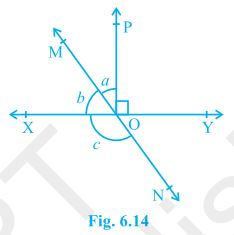Given,
∠POY = 90° and a : b = 2 : 3
A/q,
∠POY + a + b = 180°
⇒ 90° + a + b = 180°
⇒ a + b = 90°
Let a be 2x then will be 3x
2x + 3x = 90°
⇒ 5x = 90°
⇒ x = 18°
∴ a = 2×18° = 36°
and b = 3×18° = 54°
also,
b + c = 180° (Linear Pair)
⇒ 54° + c = 180°
⇒ c = 126°

3. In Fig. 6.15, ∠PQR = ∠PRQ, then prove that ∠PQS = ∠PRT.Given,
∠PQR = ∠PRQ
To prove,
∠PQS = ∠PRT
A/q,
∠PQR +∠PQS = 180° (Linear Pair)
⇒ ∠PQS = 180° - ∠PQR --- (i)
also,
∠PRQ +∠PRT = 180° (Linear Pair)
⇒ ∠PRT = 180° - ∠PRQ
⇒ ∠PRQ = 180° - ∠PQR --- (ii) (∠PQR = ∠PRQ)
From (i) and (ii)
∠PQS = ∠PRT = 180° - ∠PQR
Therefore,  ∠PQS = ∠PRT

4. In Fig. 6.16, if x + y = w + z, then prove that AOB is a line.Given,
x + y = w + z
To Prove,
AOB is a line or x + y = 180° (linear pair.)
A/q,
x + y + w + z = 360° (Angles around a point.)
⇒ (x + y) +  (w + z) = 360°
⇒ (x + y) +  (x + y) = 360° (Given x + y = w + z)
⇒ 2(x + y) = 360°
⇒ (x + y) = 180°
Hence, x + y makes a linear pair. Therefore, AOB is a staright line.

5. In Fig. 6.17, POQ is a line. Ray OR is perpendicular to line PQ. OS is another ray lying between rays OP and OR. Prove that ∠ROS = 1/2(∠QOS – ∠POS).Given,
OR is perpendicular to line PQ
To prove,
∠ROS = 1/2(∠QOS – ∠POS)
A/q,
∠POR = ∠ROQ = 90° (Perpendicular)
∠QOS = ∠ROQ ∠ROS = 90° + ∠ROS --- (i)
∠POS = ∠POR - ∠ROS = 90° - ∠ROS --- (ii)
Subtracting (ii) from (i)
∠QOS - ∠POS = 90° + ∠ROS - (90° - ∠ROS)
⇒ ∠QOS - ∠POS = 90° + ∠ROS - 90° + ∠ROS
⇒ ∠QOS - ∠POS = 2∠ROS
⇒ ∠ROS = 1/2(∠QOS – ∠POS)
Hence, Proved.

6. It is given that ∠XYZ = 64° and XY is produced to point P. Draw a figure from the given information. If ray YQ bisects ∠ZYP, find ∠XYQ and reflex ∠QYP.

Given,
∠XYZ = 64°
YQ bisects ∠ZYP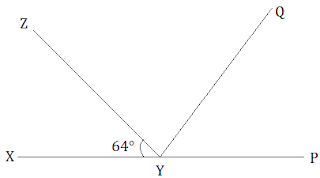∠XYZ +∠ZYP = 180° (Linear Pair)
⇒ 64° +∠ZYP = 180°
⇒ ∠ZYP = 116°
also, ∠ZYP = ∠ZYQ + ∠QYP
∠ZYQ = ∠QYP (YQ bisects ∠ZYP)
⇒ ∠ZYP = 2∠ZYQ
⇒ 2∠ZYQ = 116°
⇒ ∠ZYQ = 58° = ∠QYP
Now,
∠XYQ = ∠XYZ + ∠ZYQ
⇒ ∠XYQ = 64° + 58°
⇒ ∠XYQ = 122°
also,
reflex ∠QYP = 180° + ∠XYQ
∠QYP = 180° + 122°
⇒ ∠QYP = 302°

Page No: 103

Exercise 6.2

1. In Fig. 6.28, find the values of x and y and then show that AB || CD.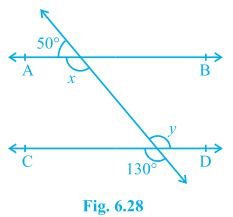x + 50° = 180° (Linear pair)
⇒ x = 130°
also,
y = 130° (Vertically opposite)
Now,
x = y = 130° (Alternate interior angles)
Alternate interior angles are equal.
Therefore, AB || CD.

Page No: 104

2. In Fig. 6.29, if AB || CD, CD || EF and y : z = 3 : 7, find x.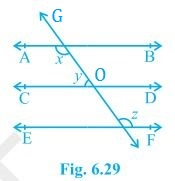Given,
AB || CD and CD || EF
y : z = 3 : 7
Now,
x + y = 180° (Angles on the same side of transversal.)
also,
∠O = z (Corresponding angles)
and, y ∠O = 180° (Linear pair)
⇒ y + z = 180°
A/q,
y = 3w and z = 7w
3w + 7w = 180°
⇒ 10 w = 180°
⇒ w = 18°
∴ y = 3×18° = 54°
and, z = 7×18° = 126°
Now,
x + y = 180°
⇒ x + 54° = 180°
⇒ x = 126°

3. In Fig. 6.30, if AB || CD, EF ⊥ CD and ∠GED = 126°, find ∠AGE, ∠GEF and ∠FGE.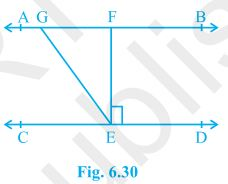Given,
AB || CD
EF ⊥ CD
∠GED = 126°
A/q,
∠FED = 90° (EF ⊥ CD)
Now,
∠AGE = ∠GED (Since, AB || CD and GE is transversal. Alternate interior angles.)
∴ ∠AGE = 126°
Also, ∠GEF = ∠GED - ∠FED
⇒ ∠GEF = 126° - 90°
⇒ ∠GEF = 36°
Now,
∠FGE +∠AGE = 180° (Linear pair)
⇒ ∠FGE = 180° - 126°
⇒ ∠FGE = 54°

4. In Fig. 6.31, if PQ || ST, ∠PQR = 110° and ∠RST = 130°, find ∠QRS.
[Hint : Draw a line parallel to ST through point R.]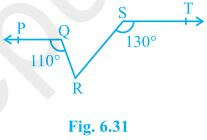Given,
PQ || ST, ∠PQR = 110° and ∠RST = 130°
Construction,
A line XY parallel to PQ and ST is drawn.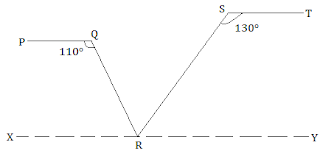∠PQR + ∠QRX = 180° (Angles on the same side of transversal.)
⇒ 110° + ∠QRX = 180°
⇒ ∠QRX = 70°
Also,
∠RST + ∠SRY = 180° (Angles on the same side of transversal.)
⇒ 130° + ∠SRY = 180°
⇒ ∠SRY = 50°
Now,
∠QRX +∠SRY ∠QRS = 180°
⇒ 70° + 50° + ∠QRS = 180°
⇒ ∠QRS = 60°

5. In Fig. 6.32, if AB || CD, ∠APQ = 50° and ∠PRD = 127°, find x and y.Given,
AB || CD, ∠APQ = 50° and ∠PRD = 127°
A/q,
x = 50° (Alternate interior angles.)
∠PRD + ∠RPB = 180° (Angles on the same side of transversal.)
⇒ 127° + ∠RPB = 180°
⇒ ∠RPB = 53°
Now,
y + 50° + ∠RPB = 180° (AB is a straight line.)
⇒ y + 50° + 53° = 180°
⇒ y + 103° = 180°
⇒ y = 77°

6. In Fig. 6.33, PQ and RS are two mirrors placed parallel to each other. An incident ray AB strikes the mirror PQ at B, the reflected ray moves along the path BC and strikes the mirror RS at C and again reflects back along CD. Prove that AB || CD.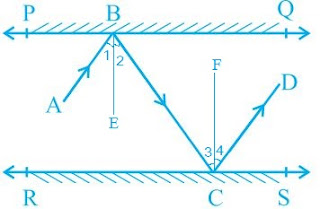Let us draw BE ⟂ PQ and CF ⟂ RS.
As PQ || RS
So, BE || CF
By laws of reflection we know that,
Angle of incidence = Angle of reflection
Thus, ∠1 = ∠2 and ∠3 = ∠4  --- (i)
also, ∠2 = ∠3     (alternate interior angles because BE || CF and a transversal line BC cuts them at B and C)    --- (ii)
From (i) and (ii),
∠1 + ∠2 = ∠3 + ∠4
⇒ ∠ABC = ∠DCB
⇒ AB || CD      (alternate interior angles are equal)

Page No: 107

Exercise 6.3

1. In Fig. 6.39, sides QP and RQ of ΔPQR are produced to points S and T respectively. If ∠SPR = 135° and ∠PQT = 110°, find ∠PRQ.Given,
∠SPR = 135° and ∠PQT = 110°
A/q,
∠SPR +∠QPR = 180° (SQ is a straight line.)
⇒ 135° +∠QPR = 180°
⇒ ∠QPR = 45°
also,
∠PQT +∠PQR = 180° (TR is a straight line.)
⇒ 110° +∠PQR = 180°
⇒ ∠PQR = 70°
Now,
∠PQR +∠QPR + ∠PRQ = 180° (Sum of the interior angles of the triangle.)
⇒ 70° + 45° + ∠PRQ = 180°
⇒ 115° + ∠PRQ = 180°
⇒ ∠PRQ = 65°

2. In Fig. 6.40, ∠X = 62°, ∠XYZ = 54°. If YO and ZO are the bisectors of ∠XYZ and ∠XZY respectively of Δ XYZ, find ∠OZY and ∠YOZ.Given,
∠X = 62°, ∠XYZ = 54°
YO and ZO are the bisectors of ∠XYZ and ∠XZY respectively.
A/q,
∠X +∠XYZ ∠XZY = 180° (Sum of the interior angles of the triangle.)
⇒ 62° + 54° + ∠XZY = 180°
⇒ 116° + ∠XZY = 180°
⇒ ∠XZY = 64°
Now,
∠OZY = 1/2∠XZY (ZO is the bisector.)
⇒ ∠OZY = 32°
also,
∠OYZ = 1/2∠XYZ (YO is the bisector.)
⇒ ∠OYZ = 27°
Now,
∠OZY +∠OYZ ∠O = 180° (Sum of the interior angles of the triangle.)
⇒ 32° + 27° + ∠O = 180°
⇒ 59° + ∠O = 180°
⇒ ∠O = 121°

3. In Fig. 6.41, if AB || DE, ∠BAC = 35° and ∠CDE = 53°, find ∠DCE.Given,
AB || DE, ∠BAC = 35° and ∠ CDE = 53°
A/q,
∠BAC = ∠CED (Alternate interior angles.)
∴ ∠CED = 35°
Now,
∠DCE +∠CED + ∠CDE = 180° (Sum of the interior angles of the triangle.)
⇒ ∠DCE + 35° + 53° = 180°
⇒ ∠DCE + 88° = 180°
⇒ ∠DCE = 92°

4. In Fig. 6.42, if lines PQ and RS intersect at point T, such that ∠PRT = 40°, ∠RPT = 95° and ∠TSQ = 75°, find ∠SQT.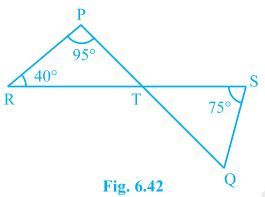Given,
∠PRT = 40°, ∠RPT = 95° and ∠TSQ = 75°
A/q,
∠PRT +∠RPT + ∠PTR = 180° (Sum of the interior angles of the triangle.)
⇒ 40° + 95° + ∠PTR = 180°
⇒ 40° + 95° + ∠PTR = 180°
⇒ 135° + ∠PTR = 180°
⇒ ∠PTR = 45°
∠PTR = ∠STQ = 45° (Vertically opposite angles.)
Now,
∠TSQ +∠PTR ∠SQT = 180° (Sum of the interior angles of the triangle.)
⇒ 75° + 45° + ∠SQT = 180°
⇒ 120° + ∠SQT = 180°
⇒ ∠SQT = 60°

Page No: 108

5. In Fig. 6.43, if PQ ⊥ PS, PQ || SR, ∠SQR = 28° and ∠QRT = 65°, then find the values of x and y.Given,
PQ ⊥ PS, PQ || SR, ∠SQR = 28° and ∠QRT = 65°
A/q,
x +∠SQR = ∠QRT (Alternate angles  as QR is transveersal.)
⇒ x + 28° = 65°
⇒ x = 37°
also,
∠QSR = x
⇒ ∠QSR = 37°
also,
∠QRS +∠QRT = 180° (Linea pair)
⇒ ∠QRS + 65° = 180°
⇒ ∠QRS = 115°
Now,
∠P ∠Q+ ∠R +∠S = 360° (Sum of the angles in a quadrilateral.)
⇒ 90° + 65° + 115° + ∠S = 360°
⇒ 270° + y + ∠QSR = 360°
⇒ 270° + y + 37° = 360°
⇒ 307° + y = 360°
⇒ y = 53°

6. In Fig. 6.44, the side QR of ΔPQR is produced to a point S. If the bisectors of ∠PQR and ∠PRS meet at point T, then prove that ∠QTR = 1/2∠QPR.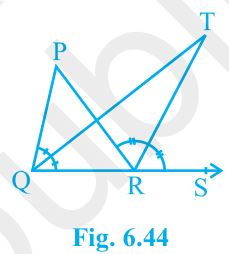Given,
Bisectors of ∠PQR and ∠PRS meet at point T.
To prove,
∠QTR = 1/2∠QPR.
Proof,
∠TRS = ∠TQR +∠QTR (Exterior angle of a triangle equals to the sum of the two interior angles.)
⇒ ∠QTR = ∠TRS - ∠TQR --- (i)
also,
∠SRP = ∠QPR ∠PQR
⇒ 2∠TRS = ∠QPR + 2∠TQR
⇒ ∠QPR =  2∠TRS - 2∠TQR
⇒ 1/2∠QPR =  ∠TRS - ∠TQR --- (ii)
Equating (i) and (ii)
∠QTR - ∠TQR = 1/2∠QPR
Hence proved.